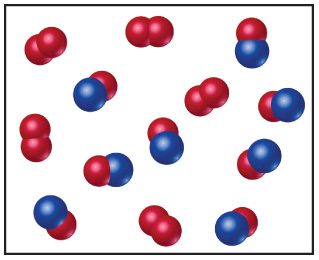# Problem: Nitrogen monoxide and oxygen react to form nitrogen dioxide. Consider the mixture of NO and O2 shown in the accompanying diagram. The blue spheres represent N, and the red ones represent O.If the actual yield of the reaction was 75% instead of 100%, how many molecules of NO2 would be present after the reaction was over?

###### FREE Expert Solution

We’re being asked to calculate the actual yield of NO2 present after the reaction.

Recall: The actual yield represents the amount of product that is made, isolate or produced when doing the reaction in real life. It can be calculated from the percent yield formula.

Before you can calculate your actual yield you must first use stoichiometry and a balanced equation to determine the theoretical yield of the limiting reactant.

89% (268 ratings)###### Problem Details

Nitrogen monoxide and oxygen react to form nitrogen dioxide. Consider the mixture of NO and O2 shown in the accompanying diagram. The blue spheres represent N, and the red ones represent O.If the actual yield of the reaction was 75% instead of 100%, how many molecules of NO2 would be present after the reaction was over?